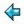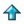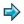### selflines.m

```output = concat[l++"\n"|l<-selflines]

selflines = mklines 1

mklines n = ("the "++ord n++" line is:"):
("\""++selflines!(n-1)++"\""):
mklines(n+1)

ord n = show n++suffix n

suffix 1 = "st"
suffix 2 = "nd"
suffix 3 = "rd"
suffix n = "th",             if n=0 \/ 3<=n&n<=9
= "th",             if (n mod 100)div 10 = 1 ||because of 11,12,13
= suffix(n mod 10), otherwise
```

This produces an endless self describing scroll of lines, as follows

```      the 1st line is
"the 1st line is"
the 2nd line is
""the 1st line is""
the 3rd line is
"the 2nd line is"
etc...
```
To see the result, say
```      output
```
Hit control-C (interrupt) when you have seen enough. If you would like to send the output to a file, say
```      output &> fil
```
where `fil' is the name of the file in which you want to put it. This will create a background job